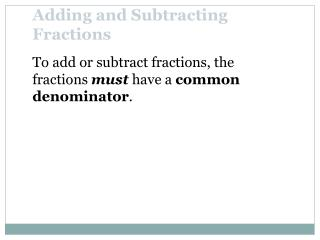DownloadDownload PresentationTo add or subtract fractions, the fractions must have a common denominator .

# To add or subtract fractions, the fractions must have a common denominator .

Télécharger la présentation## To add or subtract fractions, the fractions must have a common denominator .

- - - - - - - - - - - - - - - - - - - - - - - - - - - E N D - - - - - - - - - - - - - - - - - - - - - - - - - - -
##### Presentation Transcript

1. Adding and Subtracting Fractions To add or subtract fractions, the fractions must have a common denominator.

2. Fractions that have the same or common denominator are called like fractions.Fractions that have different denominators are called unlike fractions. Like and Unlike Fractions Like Fractions Unlike Fractions

3. Adding or Subtracting Like Fractions If a, b, and c, are numbers and b is not 0, then To add or subtract fractions with the same denominator, add or subtract their numerators and write the sum or difference over the common denominator.

4. Least Common Denominator To add or subtract fractions that have unlike, or different, denominators, we write the fractions as equivalent fractions with a common denominator. The smallest common denominator is called the least common denominator (LCD) or the least common multiple (LCM).

5. Least Common Multiple The least common denominator (LCD) of a list of fractions is the smallest positive number divisible by all the denominators in the list. (The least common denominator is also the least common multiple (LCM)of the denominators.)

6. Least Common Denominator To find the LCD of First, write each denominator as a product of primes. 12 = 2 • 2 • 3 18 = 2 • 3 • 3 Then write each factor the greatest number of times it appears in any one prime factorization. The greatest number of times that 2 appears is 2 times. The greatest number of times that 3 appears is 2 times. LCD = 2 • 2 • 3 • 3 = 36

7. Examples Simplify: